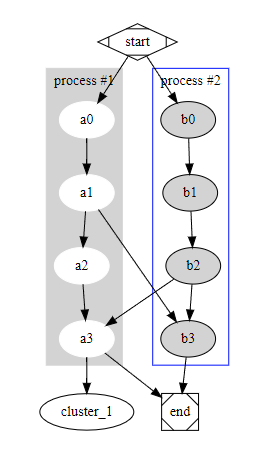# Can a node point to a cluster without involving that cluster own nodes?

I am considering moving from mermaid.js to graphviz but one point is still blocking me: the ability for a node to point to a cluster.

Taking the typical example everyone shows (the version, modified with my attempts is online), I tried to point the node `a3` to the cluster `cluster_1`:

``````digraph G {
compount=true;

subgraph cluster_0 {
style=filled;
color=lightgrey;
node [style=filled,color=white];
a0 -> a1 -> a2 -> a3;
label = "process #1";
}

subgraph cluster_1 {
node [style=filled];
b0 -> b1 -> b2 -> b3;
label = "process #2";
color=blue
}
start -> a0;
start -> b0;
a1 -> b3;
b2 -> a3;
a3 -> cluster_1;
a3 -> end;
b3 -> end;

start [shape=Mdiamond];
end [shape=Msquare];
}
``````

The result is`cluster_1` is perceived in that context as a new node instead of the cluster labeled `process #2`.

Is there a way to have a node point to a cluster, without having it point to a node in that cluster? The emphasized part is important because I just know that a node points to a cluster, (and not a node in that cluster).

In other words, I cannot do, for instance,

``````a3 -> b0 [lhead=cluster_1];
``````

(because that requires me to know that `b0` is part of `cluster_1`)

A post was merged into an existing topic: About the Help category

I don’t know if the dot layout engine supports edges to/from clusters, but fdp does. See this example.

Only fdp supports clusters as head and/or tail of an edge (though this feature was recently broken - see fdp cluster->cluster edges were correct but now drawn incorrectly (#2345) · Issues · graphviz / graphviz · GitLab)

Long answer & (lightly tested) work-around

Below is a (very lightly tested) gvpr (part of the Graphviz bundle) (see http://www.graphviz.org/pdf/gvpr.1.pdf) program that will take an input file containing edges with clusters as head and/or tail and produce a modified result, suitable for input into dot, with compound=true and each cluster-involved edge recreated as an edge to/from one of the nodes contained within the cluster.

The gvpr program:

``````BEGIN{
graph_t aGraph, Root, Parent[];
node_t  aNode, newH, newT, theKid[], kid[];
edge_t  newE, anEdge;
int     i, K, clust[], Clust[], rankCnt[], maxRnk[], deleteE[], deleteN[];

graph_t graphTraverse(graph_t thisG){
for (aGraph = fstsubg(thisG); aGraph; aGraph = nxtsubg(aGraph)) {
//print ("//  graph:  >", aGraph.name,"<");
if (match(aGraph.name,"cluster")==0 || (hasAttr(aGraph, "cluster") && aGraph.cluster=="true")){
clust[aGraph.name]=1;  // ?????
Clust[aGraph]=1;
print ("// CLUSTER ",aGraph.name);
K=0;
for (aNode=fstnode(aGraph);aNode;aNode = nxtnode_sg(aGraph, aNode)){
Parent[aNode]=aGraph;
kid[++K]=aNode;
}
i=(int)(K/2);  // or we could randomize??
theKid[aGraph]=kid[i];
}
aGraph = graphTraverse(aGraph);
}
return thisG;
}  // end of graphTraverse
}
BEG_G {
Root=\$G;
graphTraverse (\$G);
}
E{
print("// ", \$.name);
newT=\$.tail;
\$G.compound="true";
deleteE[\$]=1;
}
if (clust[\$.tail.name]==1){
print ("// BINGO - tail ",\$.tail.name);
\$G.compound="true";
deleteE[\$]=1;
newT=theKid[\$.tail];
}
print("// NEW: ", newT.name, "  ", newH.name);
newE=edge(newT, newH,"");
copyA(\$, newE);
if (newT != \$.tail){
newE.ltail=\$.tail.name;
deleteN[\$.tail]=1;
}
`gvpr -cf clusterCluster.gvpr myFile.gv | dot -Tpng >myFile.png`